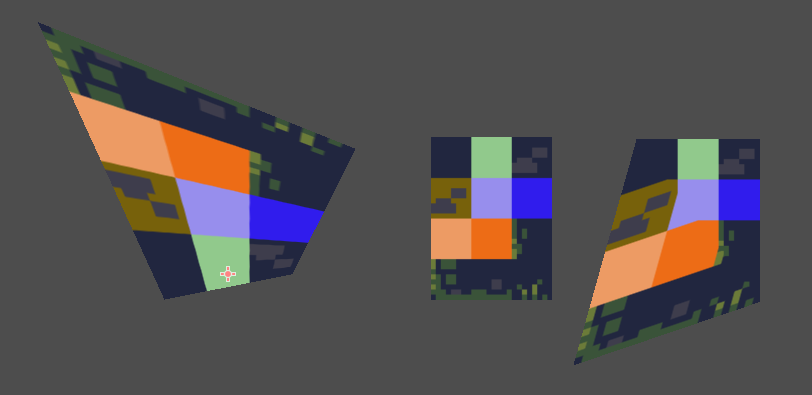I am trying to make a shader to warp an image in godot, but I only seem to create uniform skews and all tutorials just show me how to make things wiggle. How can I make the can accomplish what I have in the image below?Godot version 3.2.3
in Engine

``````shader_type canvas_item;
render_mode blend_mix;

uniform float heightOffset = 0.3;
uniform float playerSpeed = 0;

void vertex() {
VERTEX.x += max(0.0, (1.0-UV.y) - heightOffset) * (playerSpeed / 12.0);
VERTEX.y += max(0.0, (UV.x / 2.5) - heightOffset) * (playerSpeed / 12.0);
}
``````

Any progress? Is my answer helping?

We have to displace the vertices in pixels.

Having a MeshInstance2D (100,100) in size I got this to work when subdivide (10,10) enough to remove the triangle wiggles.``````shader_type canvas_item;

// naming in UV system _x_y
uniform vec2 offset_0_0 = vec2(0.0, 0.0);
uniform vec2 offset_0_1 = vec2(0.0, 0.0);
uniform vec2 offset_1_0 = vec2(0.0, 0.0);
uniform vec2 offset_1_1 = vec2(0.0, 0.0);

vec2 sample_point(vec2 point) {
// Corners for UV.y == 0
vec2 p_0_0 = vec2(0,0) - offset_0_0;
vec2 p_1_0 = vec2(1,0) - offset_1_0;
// Get point along line UV.y == 0
vec2 d_x_0 = p_0_0 + (p_1_0 - p_0_0) * point.x;

// Corners for UV.y == 1.0
vec2 p_0_1 = vec2(0, 1) - offset_0_1;
vec2 p_1_1 = vec2(1, 1) - offset_1_1;
// Get point along line UV.y == 1
vec2 d_x_1 = p_0_1 + (p_1_1 - p_0_1) * point.x;
// Get point for given UV.y
return d_x_0 + (d_x_1 - d_x_0) * point.y;
}

void fragment() {
//COLOR = vec4(UV.x, UV.y, .0, 1.0);
//COLOR = texture(TEXTURE, UV);
//COLOR = texture(TEXTURE, sample_point(UV));
}

void vertex() {
VERTEX += sample_point(UV);
}
``````

I've kept the fragment code as we can distort using `fragment()` only. This requires to comment out the vertex call and scale offsets in range [0,1]

Using a sprite does not work as that has 2 triangles for its quad so there is big distortion. For that to work I guess sample_point must be split into _fragment and _vertex variant.by (646 points)
edited

I can't find a way to use PlaneMesh in MeshInstance2D since the default orientation of plane mesh is along X and Z axis.

And QuadMesh doesn't provide a subdivision option.

What is the solution for 2D?